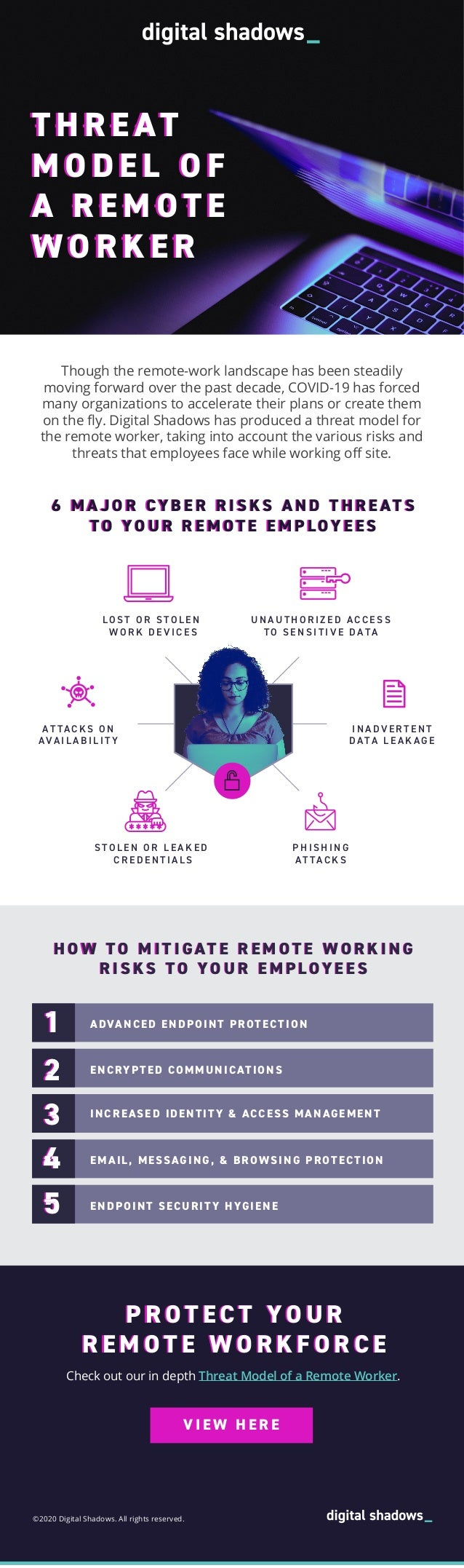Successfully reported this slideshow.
We use your LinkedIn profile and activity data to personalize ads and to show you more relevant ads. You can change your ad preferences anytime.Upcoming SlideShare
Loading in …5
×

# Threat model of a remote worker | Infographic

Though the remote-work landscape has been steadily moving forward over the past decade, COVID-19 (aka coronavirus) has forced many organizations to accelerate their plans or create them on the fly. As such, Digital Shadows has produced a threat model for the remote worker, taking into account the various risks and threats that employees face while working off site.

• Full Name
Comment goes here.

Are you sure you want to Yes No
Your message goes here• Be the first to comment

### Threat model of a remote worker | Infographic

1. 1. H O W T O M I T I GAT E R E M O T E W O R K I N G R I S KS T O YO U R E M P LOY E E S T H R E AT M O D E L O F A R E M O T E W O R K E R T H R E AT M O D E L O F A R E M O T E W O R K E R T H R E AT M O D E L O F A R E M O T E W O R K E R T H R E AT M O D E L O F A R E M O T E W O R K E R T H R E AT M O D E L O F A R E M O T E W O R K E R T H R E AT M O D E L O F A R E M O T E W O R K E R T H R E AT M O D E L O F A R E M O T E W O R K E R T H R E AT M O D E L O F A R E M O T E W O R K E R A DVA N C E D E N D P O I N T P R O T E C T I O NENCRYPTED COMMUNICATIONS ADVANCED ENDPOINT PROTECTION A DVA N C E D E N D P O I N T P R O T E C T I O NEMAIL, MESSAGING, & BROWSING PROTECTION INCREASED IDENTITY & ACCESS MANAGEMENT ENDPOINT SECURITY HYGIENE 11 22 55 11 2222222222 3333 4444 55 Check out our in depth Threat Model of a Remote Worker. P R O T E C T Y O U R R E M O T E W O R K F O R C E ©2020 Digital Shadows. All rights reserved. V I E W H E R E H O W T O M I T I GAT E R E M O T E W O R K I N G R I S KS T O YO U R E M P LOY E E S Though the remote-work landscape has been steadily moving forward over the past decade, COVID-19 has forced many organizations to accelerate their plans or create them on the ﬂy. Digital Shadows has produced a threat model for the remote worker, taking into account the various risks and threats that employees face while working oﬀ site. P R O T E C T Y O U R R E M O T E W O R K F O R C E 6 M A J O R CY B E R R I S KS A N D T H R E AT S T O YO U R R E M O T E E M P LOY E E S LO S T O R S T O L E N W O R K D E V I C E S S T O L E N O R L E A K E D C R E D E N T I A L S AT TA C K S O N AVA I L A B I L I T Y U N A U T H O R I Z E D A C C E S S T O S E N S I T I V E D ATA P H I S H I N G AT TA C K S I N A D V E R T E N T D ATA L E A K A G E 6 M A J O R CY B E R R I S KS A N D T H R E AT S T O YO U R R E M O T E E M P LOY E E S